## A car of 900 kg mass is moving at the velocity of 60 km/hr. It is brought into rest at 50 meter distance by applying a brake. Now, calculate

Question

A car of 900 kg mass is moving at the velocity of 60 km/hr. It is brought into rest at 50 meter distance by applying a brake. Now, calculate the force required to stop the car.

in progress 0
6 months 2021-09-05T00:32:14+00:00 1 Answers 0 views 0

1. Answer: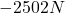Explanation: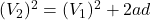where;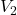= final velocity = 0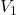= initial velocity = 60 km/h = 16.67 m/s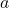= acceleration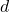= distance

First all of, because acceleration is given in m/s and not km/h, you need to convert 60km/h to m/s. Our conversion factors here are 1km = 1000m and 1h = 3600s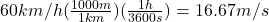Solve for a;Begin by subtracting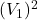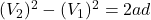Divide by 2d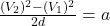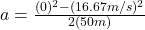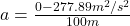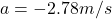If you’re wondering why I calculated acceleration first is because in order to find force, we need 2 things: mass and acceleration.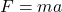m = mass = 900kg

a = acceleration = -2.78m/s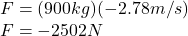It’s negative because the force has to be applied in the opposite direction that the car is moving.